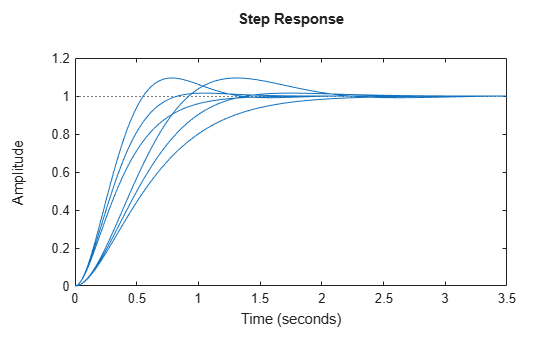Main Content

# Study Parameter Variation by Sampling Tunable Model

This example shows how to sample a parametric model of a second-order filter across a grid of parameter values using `sampleBlock`.

Consider the second-order filter represented by:

`$F\left(s\right)=\frac{{\omega }_{n}^{2}}{{s}^{2}+2\zeta {\omega }_{n}s+{\omega }_{n}^{2}}.$`

Sample this filter at varying values of the damping constant $\zeta$ and the natural frequency ${\omega }_{n}$. Create a parametric model of the filter by using tunable elements for $\zeta$ and ${\omega }_{n}$.

```wn = realp('wn',3); zeta = realp('zeta',0.8); F = tf(wn^2,[1 2*zeta*wn wn^2])```
```F = Generalized continuous-time state-space model with 1 outputs, 1 inputs, 2 states, and the following blocks: wn: Scalar parameter, 5 occurrences. zeta: Scalar parameter, 1 occurrences. Type "ss(F)" to see the current value, "get(F)" to see all properties, and "F.Blocks" to interact with the blocks. ```

`F` is a `genss` model with two tunable Control Design Blocks, the `realp` blocks `wn` and `zeta`. The blocks `wn` and `zeta` have initial values of 3 and 0.8, respectively.

Sample `F` over a 2-by-3 grid of (`wn`, `zeta`) values.

```wnvals = [3;5]; zetavals = [0.6 0.8 1.0]; Fsample = sampleBlock(F,'wn',wnvals,'zeta',zetavals);```

Here, `sampleBlock` samples the model independently over the two ${\omega }_{n}$ values and three $\zeta$ values. Thus, `Fsample` is a 2-by-3 array of state-space models. Each entry in the array is a state-space model that represents `F` evaluated at the corresponding (`wn`, `zeta`) pair. For example, `Fsample(:,:,2,3)` has `wn` = 5 and `zeta` = 1.0.

Set the `SamplingGrid` property of the model array to help keep track of which set of parameter values corresponds to which entry in the array. To do so, create a grid of parameter values that matches the dimensions of the array. Then, assign these values to `Fsample.SamplingGrid` in a structure with the parameter names.

```[wngrid,zetagrid] = ndgrid(wnvals,zetavals); Fsample.SamplingGrid = struct('wn',wngrid,'zeta',zetagrid);```

The `ndgrid` command produces the full 2-by-3 grid of (`wn`, `zeta`) combinations. When you display `Fsample` in the command window, the parameter values in `Fsample.SamplingGrid` are displayed along with the each transfer function in the array. The parameter information is also available in response plots. For instance, examine the step response of `Fsample`.

`stepplot(Fsample)`The step response plots show the variation in the natural frequency and damping constant across the six models in the array. When you click on one of the responses in the plot, the datatip includes the corresponding `wn` and `zeta` values as specified in `Fsample.SamplingGrid`.

## Support

#### Learn how to automatically tune PID controller gains

Download code examples